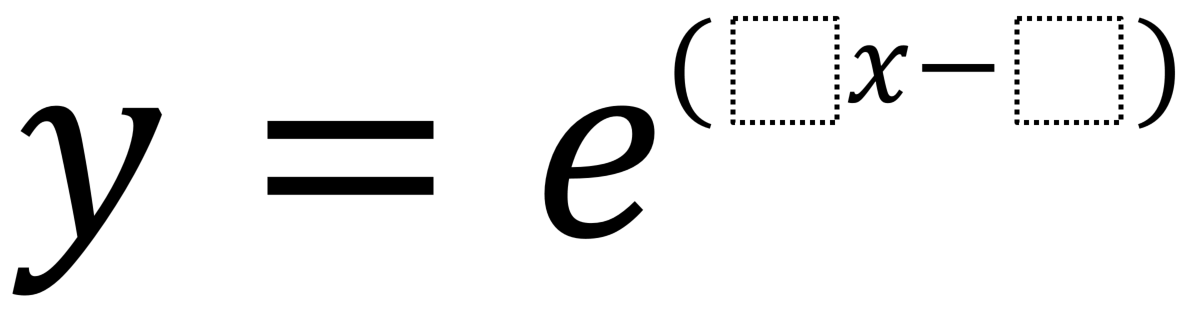Home > Calculus > Derivative of e

# Derivative of e

Directions: Using the digits 1-6, at most one time each, create an exponential function of base e whose derivative at x = 3 is 2.### Hint

What is the process for finding the derivative?
What will make e^x ‘disappear’?

### Answer

y=e^(2x-6)

Source: Christine Relleva

## Derivative of Trig Functions 2

Directions: Fill in the boxes below using the digits 1 to 6, at most one …

### One comment

1.Hi Christine! I think you need a factor of 2 outside the exponential function like 2e^(x-3). As a follow-up you might ask students to check g'(3) if
g(x)= (2/e^3)•e^x. Then ask, “Are the solutions equivalent?”. Keep writing these great problems!😊
Dave